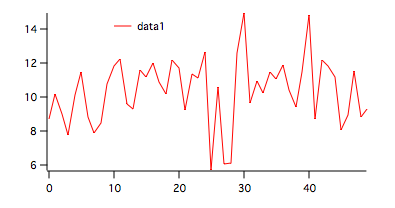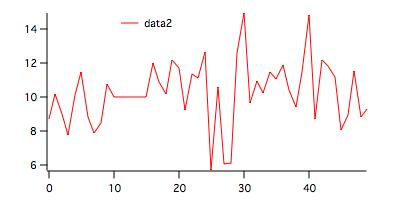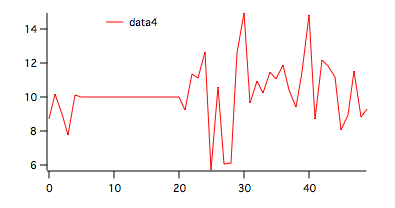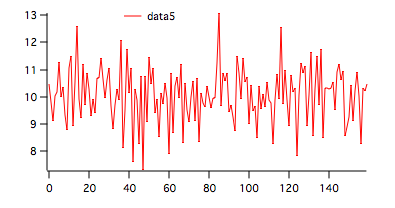The StatsSRTest operation contains both parametric and non-parametric serial randomness tests. In the following examples we illustrate how they work (and how they fail).

1. Testing serial randomenss of gnoise().

The first data set is created with the command:

`Make/n=50 data1=10+gnoise(2)`

The resulting wave appears in the figure below.To run both tests on this wave execute the command:

`StatsSRTest/T=1/Q/P/NP  data1`

The results are displayed in the Serial Randomness Test table:

 N 50 s2 3.71269 ss2 3.30635 C 0.109447 Critical 0.228249 P-Value 0.217518 Runs Up 17 Runs Down 16 Unchanged 0 Longest Run 4 Longest Run Prob. 0.473586 Total Runs 33 Prob 0.565534

The first six rows in the table belong to the parametric test. In this case we have a statistic C which is smaller than the critical value so the hypothesis H0 that the wave contains a random variation is accepted though the P-value is not particularly high. The remaining rows of the table belong to the non-parametric test. After listing the number of runs up and down, the table includes the longest run and the probability that a run of such length can happen by chance. The last two rows contain the total number of runs and the probability that such a value can be attained by chance.

2. Testing serial randomness in the presence of non-random data segment.

We create the second data set by duplicating the first and setting a contiguous run of length N/10 to a constant. The following commands were used:

`Duplicate data1,data2`
`data2[10,14]=10`To run both tests on this wave execute:

`StatsSRTest/T=1/Q/P/NP  data2`

The results are displayed in the Serial Randomness Test table:

 N 50 s2 3.53478 ss2 3.18483 C 0.099004 Critical 0.228249 P-Value 0.240177 Runs Up 16 Runs Down 15 Unchanged 1 Longest Run 4 Longest Run Prob. 0.473586 Converted 0 Total Runs 31 Prob 0.302833

The results of the parametric test are similar to those of the test in (1) above. The non-parametric tests shows a slightly lower probability of attaining 31 runs by chance but this value is still not enough to reject H0.

3. Testing serial randomness in the presence of a non-random segment set to be different from the mean value.

`Duplicate data1,data3`
`data3[10,14]=0`To run both tests on this wave execute:

`StatsSRTest/T=1/Q/P/NP  data3`

The results are displayed in the Serial Randomness Test table:

 N 50 s2 13.268 ss2 5.61904 C 0.576496 Critical 0.228249 P-Value 3.07736e-06 Runs Up 16 Runs Down 15 Unchanged 1 Longest Run 4 Longest Run Prob. 0.473586 Converted 0 Total Runs 31 Prob 0.302833

As expected, the non-parametric results remain unchanged but the parametric test statistic C exceeds the critical value and so H0 must be rejected. This illustrates a major deficiency of the parametric test in that it is less likely to fail if the non-random sections have values sufficiently close to the mean.

4. Testing in the presence of a very long non-random segment.

We used the following commands to create the input wave:

`Duplicate data1,data4`
`data4[5,20]=10`To run both tests on this wave execute:

`StatsSRTest/T=1/Q/P/NP  data4`

The results are displayed in the Serial Randomness Test table:

 N 50 s2 3.08189 ss2 2.88656 C 0.063382 Critical 0.228249 P-Value 0.325941 Runs Up 13 Runs Down 13 Unchanged 1 Longest Run 15 Longest Run Prob. 3.15448e-12 Converted 1 Max Runs 27 Min Runs 25 Mean Runs 26 Max Prob 0.0303374 Min Prob 0.005209 Mean Prob 0.005209

In this case, despite the presence of a very long constant segment, the parametric test does not reject H0. On the other hand, the non-parametric test indicates a vanishing probability of the longest run being that long by chance and (all) run probabilities are too low to accept H0.

5. Testing longer waves.

If the wave that you are testing exceeds 150 points, the parametric test uses the normal approximation to calculate the critical value and the P-value. If your wave has more than 100 points then the non-parametric test uses the normal approximation for the runs up and down distribution. For example,

`Make/O/N=160 data5=10+gnoise(1)``StatsSRTest/T=1/Q/P/NP  data5`

 N 160 s2 0.994158 ss2 1.04365 C -0.0497789 Normal Approx Z -0.631623 Critical 1.64485 Runs Up 52 Runs Down 52 Unchanged 0 Longest Run 4 Longest Run Prob. 0.885759 Total Runs 104 Normal Approx Z -0.439999 Critical 1.64485ForumSupportGallery